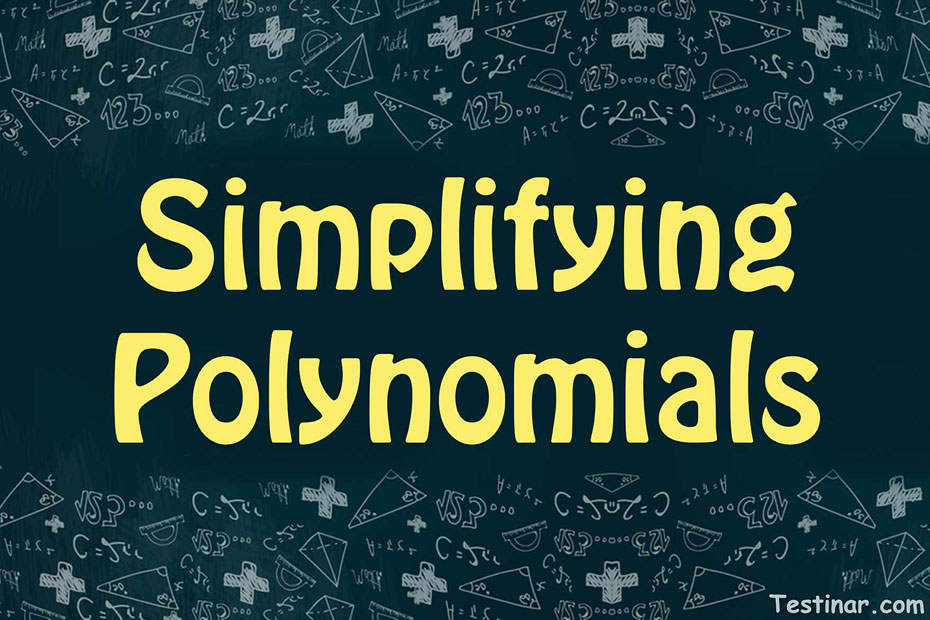## How to Simplify Polynomials

As the name suggests, the word “polynomial” can be cut into two words “poly” and “nomial” which means multiple expressions. Therefore, a polynomial refers to an algebraic or a mathematical expression that consists of one or more terms. The expressions in the polynomial terms could be a constant, a variable, or even a mixed term with a co-efficient. Also, in a polynomial, the power of the variable (let’s suppose  $$x$$) should be an integer and not a fractional power like a squared root or a cube root.
For example, $$2x^\frac{1}{3} \ + \ 3x \ + \ 1$$ is not a polynomial as the first term has a fraction power of $$x$$.

### Degree of a Polynomial Expression

To know the degree of a single variable expression, follow these steps:

• First, simplify the polynomial expression.
• Then, write the polynomial expression in the standard form.
• Next, you should check and select the highest exponent of the variable term.

Ex: $$4x^3 \ + \ 3x^2 \ + \ 7$$ has the degree $$3$$ since the highest exponent of $$x$$ is $$3$$.

To know the degree of a multivariable polynomial expression, follow these steps:

• First, simplify the polynomial expression.
• Then, write the polynomial expression in the standard form.
• Next, you should check and select the highest exponent of the variable term. Note: you should add the powers of different variables too.

Ex: $$x^3 \ + \ 3x^2y^3 \ + \ 4$$ has the degree $$5$$, since the middle term has the power of $$x \ = \ 2$$ and $$y \ = \ 3$$, so the addition comes to $$5$$.

### Simplifying Polynomial Expressions

To simplify a polynomial expression, apply the below-mentioned steps:

• First, simplify the expression by adding/subtracting the like terms.
• Also, wherever possible, use the distributive property.

Some Examples:

• $$4x^3 \ + \ 3x^3 \ + \ 2x^2 \ - \ x^2 \ + \ 9 \ = \ 7x^3 \ + \ x^2 \ + \ 9$$.
• $$2x^3 \ - \ 5x^3 \ + \ 7x^2 \ - \ x^2 \ + \ 5 \ = \ -3x^3 \ + \ 6x^2 \ + \ 5$$.
• $$-x^3 \ + \ 7x^3 \ - \ x^2 \ + \ x^2 \ + \ 7 \ = \ 6x^3 \ + \ 7$$.
• $$12x^3 \ + \ 15x^3 \ - \ 2x^2 \ - 8x^2 \ + \ 19 \ = \ 27x^3 \ - \ 10x^2 \ + \ 19$$.

### Exercises for Simplifying Polynomials

1) $$16 \ + \ 3x^3 \ - \ 7x^2 \ - \ 2 =$$

2) $$25 \ + \ 7x^3 \ - \ 5x^2 \ - \ 4 =$$

3) $$18 \ + \ 3(-2x^3 \ - \ 4x^2) \ - \ 2 + x =$$

4) $$3x (x \ + \ 5x^2 \ - \ 2x^4) =$$

5) $$18x (x \ + \ 6x^2 \ - \ 7x^4) =$$

6) $$(-x \ + \ 7x^2)x =$$

7) $$(x \ - \ 20x^2)(x \ + \ 2) =$$

8) $$(-x \ + \ 14x^2)x =$$

9) $$(x \ - \ 5x^2)(x \ + \ 3) =$$

10) $$(x \ - \ 6x^2)(x \ + \ 3) =$$

1) $$16 \ + \ 3x^3 \ - \ 7x^2 \ - \ 2 =$$$$\ \color{red}{3x^3 \ - \ 7x^2 \ + \ 14}$$
2) $$25 \ + \ 7x^3 \ - \ 5x^2 \ - \ 4 =$$$$\ \color{red}{7x^3 \ - \ 5x^2 \ + \ 21}$$
3) $$18 \ + \ 3(-2x^3 \ - \ 4x^2) \ - \ 2 + x =$$$$\ \color{red}{-6x^3 \ - \ 12x^2 \ + \ x \ + \ 16}$$
4) $$3x (x \ + \ 5x^2 \ - \ 2x^4) =$$$$\ \color{red}{-6x^4 \ + \ 15x^3 \ + \ 3x^2}$$
5) $$18x (x \ + \ 6x^2 \ - \ 7x^4) =$$$$\ \color{red}{-126x^4 \ + \ 108x^3 \ + \ 18x^2}$$
6) $$(-x \ + \ 7x^2)x =$$$$\ \color{red}{7x^3 \ - \ x^2}$$
7) $$(x \ - \ 20x^2)(x \ + \ 2) =$$$$\ \color{red}{-20x^3 \ - \ 39x^2 \ + \ 2x}$$
8) $$(-x \ + \ 14x^2)x =$$$$\ \color{red}{14x^3 \ - \ x^2}$$
9) $$(x \ - \ 5x^2)(x \ + \ 3) =$$$$\ \color{red}{-5x^3 \ - \ 14x^2 \ + \ 3x}$$
10) $$(x \ - \ 6x^2)(x \ + \ 3) =$$$$\ \color{red}{-6x^3 \ - \ 17x^2 \ + \ 3x}$$

## Simplifying Polynomials Practice Quiz

### SSAT Upper Level Math for Beginners

$24.99$14.99

### Prepare for the SHSAT Math Test in 7 Days

$17.99$12.99

### The Most Comprehensive ISEE Upper Level Math Preparation Bundle

$76.99$36.99

### Praxis Math Formulas

$6.99$5.99